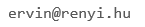﻿ BSM

## Graph Theory, Track A

• Instructor: Ervin Gyôri
• Contact:• Prerequisites: Some degree of mathematical maturity is needed for this relatively fast paced course. No prior knowledge of graph theory is required.
• Text: R. Diestel, Graph Theory (available in a digital edition, too) + handouts

Course description: A brief overview of the basic concepts of graph theory will be followed by an in-depth discussion of some classical chapters of graph theory as well as some advanced current subjects like high connectivity and extremal graph theory.

Topics:
Basics:Graphs, degrees, paths and cycles, independent sets, cliques, isomorphism, sub- graphs, complement of a graph, trees, Euler tours.
Hamilton cycles: sufficient conditions for Hamiltonicity, theorems of Dirac, Ore, P ´osa, and Chv ´atal, the optimality of Chvatal’s theorem.
Matchings: matchings in bipartite graphs, Hall’s theorem and Konig’s theorem, Tutte’s theorem, stable matchings, Gale-Shapley theorem.
Colorings: The concept of chromatic number, its relation to the clique number, Mycielsky’s construction, coloring edges, Vizing’s theorem, list coloring, choice number, its relation to the chromatic number, list coloring conjecture, Galvin’s theorem.
Planarity: Euler’s formula, Kuratowski’s theorem, coloring of planar graphs, list coloring of planar graphs.
Basics of extremal graph theory: Turan’s theorem and related results, graph Ramsey theorems.
Perfect graphs: Basic examples, perfectness of comparability graphs, the Perfect Graph Theorem, the Strong Perfect Graph Theorem.
High connectivity of graphs: Menger’s theorem, k-connected and k-linked graphs, Gy ?ori-Lov ´asz theorem on k-connected graphs, highly connected subgraphs, topological complete subgraphs.
Advanced extremal graph theory: Erdos-Gallai extremal theorem on paths, extremal theorems on cycles of given length, regularity lemma and its application to Erdos-Stone- Simonovits theorem.